# SARIMA模型在新疆布鲁氏菌病发病预测中的应用Application of SARIMA Model in Prediction of Brucellosis in Xinjiang

DOI: 10.12677/AAM.2021.104134, PDF, HTML, XML, 下载: 7  浏览: 35  科研立项经费支持

Abstract: In order to fit the new incidence of brucellosis in Xinjiang, this paper uses ARIMA(P,D,q)(P,D,Q)12 model to make short-term prediction and discusses the feasibility of the model. This paper collects the monthly incidence of human brucellosis in Xinjiang from January 2004 to December 2016, and uses R software to find the optimal model and make prediction. First, the incidence of brucellosis in the 12 months of 2017 is predicted. Secondly, the value from February to December 2016 is fitted, and compared with the actual value from February to December 2016, the ARIMA(1,1,0)(0,1,0)12 model (AIC = 1606.44) is finally established, which has higher effectiveness and rationality. The model fits the new incidence of human brucellosis in Xinjiang well, and can be used for short-term prediction and effective prevention of brucellosis.

1. 引言

2. 资料与方法

2.1. 数据来源

2.2. 模型的建立

1) 季节效应分析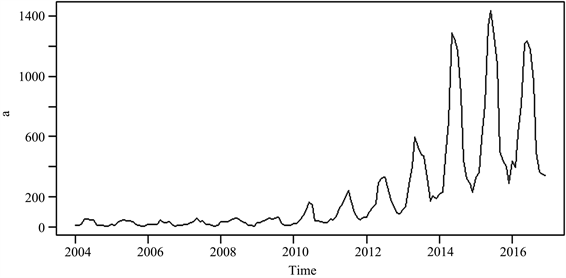Figure 1. Monthly incidence of brucellosis in Xinjiang from January 2004 to December 2016 (a)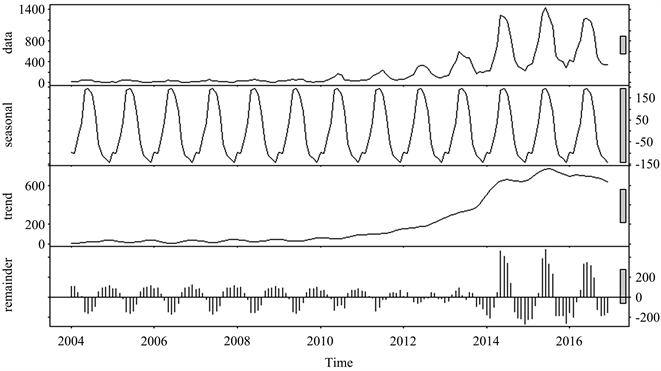Figure 2. Decomposition of seasonal factors of brucellosis in Xinjiang

2) SARIMA模型介绍

SARIMA模型：较早的文献也称其为乘积ARIMA模型，是随机季节模型与ARIMA模型的结合，对于时间序列{Z, t = 1, 2, ∙∙∙}有季节性、趋势性和周期性时，可以建立非平稳季节模型，表示为SARIMA (p,d,q)(P,D,Q)的模型，其一般形式为 ：

${\varphi }_{p}\left(L\right){\Phi }_{p}\left({L}^{s}\right){\left(1-L\right)}^{d}{\left(1-{L}^{s}\right)}^{D}{Z}_{t}={\theta }_{q}\left(L\right){\Theta }_{Q}\left({L}^{s}\right){\epsilon }_{t}$

${\varphi }_{p}\left(L\right)=1-{\varphi }_{1}L-{\varphi }_{2}{L}^{2}-\cdots -{\varphi }_{p}{L}^{p}$

${\Phi }_{P}\left({L}^{s}\right)=1-{\varphi }_{s}{L}^{s}-{\varphi }_{2s}{L}^{2s}-\cdots -{\varphi }_{Ps}{L}^{Ps}$

${\theta }_{q}\left(L\right)=1-{\theta }_{1}L-{\theta }_{2}{L}^{2}-\cdots -{\theta }_{q}{L}^{q}$

${\Theta }_{Q}\left({L}^{s}\right)=1-{\Theta }_{s}{L}^{s}-{\Theta }_{2s}{L}^{2s}-\cdots -{\Theta }_{Qs}{L}^{Qs}$

p为非季节自回归阶数，P为季节自回归阶数，q为非季节移动平均阶数，Q为季节自回归阶数。 $d,D$ 分别为普通差分和季节差分的阶数，s 为季节的长度， ${\epsilon }_{t}$ 为白噪声序列。

3) SARIMA模型建立步骤

b) 非平稳序列平稳化：根据平稳序列acf图和偏自相关系数pacf图，选择适当的阶数。

c) SARIMA模型识别，模型识别过程中为了避免因经验不足而导致的模型识别不准确问题，使用Ｒ软件auto.arima函数自动识别模型阶数，并给出模型参数 。

d) 参数估计及模型诊断与优化：运用最大似然估计，充分利用序列的信息对模型中未知参数进行估计。模型检验参数的显著性检验，当P < 0.05时可认为参数显著。通过模型检验的SARIMA(p,d,q)(P,D,Q)12模型，可采用赤则准则(AIC)，贝叶斯信息准则(BIC)确定最优模型。

e) 模型预测：选择最优模型，在80%和95%的置信区间进行短期预测。

$\text{RMSE}=\sqrt{{n}^{-1}\underset{t=1}{\overset{n}{\sum }}{\left({e}_{t}\right)}^{2}}$

$\text{MASE}={n}^{-1}\underset{t=1}{\overset{n}{\sum }}|{e}_{t}|/q$

(在MASE中，q对不同的对象有不同的意义，下面是针对季节性时间序列)

$q=\frac{1}{n-m}\underset{t=m+1}{\overset{n}{\sum }}|{x}_{t}-{x}_{t-m}|$

3. 结果

3.1. 原始序列平稳性检验

3.2. 模型识别Figure 3. Various difference graphs of the sequence of a: { $\nabla {X}_{t}$ } (top), { ${\nabla }_{12}{X}_{t}$ } (middle), { $\nabla {\nabla }_{12}{X}_{t}$ } (bottom)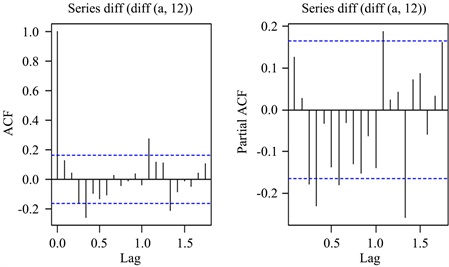Figure 4. acf and pacf graphs after first-order difference and second-order seasonal difference

3.3. 参数估计及模型诊断与优化

3.3.1. 参数估计

SARIMA(p,d,q)(P,D,Q)12模型可能的组合结果如表1所示。首先考虑建立SARIMA(2,1,2)(1,1,1)12模型，若显著性水平α = 0.1，其中变量MA(1)的t值 = −0.3605、P = 0.3595 > 0.1，SAR(1)的t值 = 0.4837、P = 0.3147 > 0.1、SMA(1)的t值 = −0.5645、P = 0.2866 > 0.1，三者都没通过t检验。然后剔除变量MA(1)、SAR(1)、SMA(1)，尝试建立SARIMA(2,1,0)(0,1,0)12模型，变量AR(2)的t值 = 0.3238、P = 0.3733 > 0.1，所以剔除AR(2)，建立SARIMA(1,1,2)(0,1,0)12，变量AR(1)、MA(1)不显著，剔除AR(1)，建立SARIMA (0,1,2)(0,1,0)12，变量MA(2)不显著，所以剔除MA(2)，建立SARIMA(0,1,1)(0,1,0)12，t检验通过，在这提一下，R语言里的auto.arima函数可以帮助我们找到合适的模型，也就是它的参数检验都通过，刚好该函数选择的模型就是SARIMA(0,1,1)(0,1,0)12，接着建立SARIMA(1,1,0)(0,1,0)  模型，t检验也通过。Table 1. Parameter estimation and model diagnosis of SARIMA model

3.3.2. 模型诊断与优化Figure 5. Residual sequence (left), density histogram and density estimation (middle), normal QQ graph (right) of SARIMA(0,1,1)(0,1,0)12 model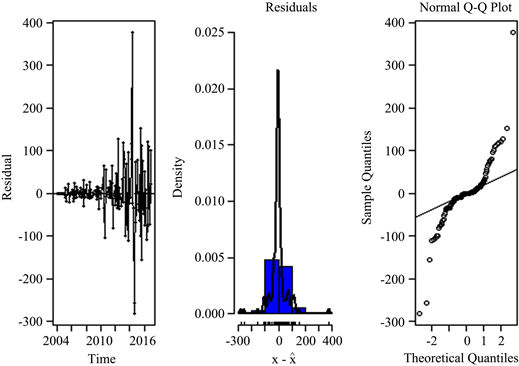Figure 6. Residual sequence (left), density histogram and density estimation (middle), normal QQ graph (right) of SARIMA(1,1,0)(0,1,0)12 modelTable 2. Selection criteria test of optimal SARIMA model

3.4. 模型预测

1) 预测2017年1月~2017年12月的新发病数

2) 2016年1月以前的数据作为训练集，2016年2月以后的数据作为测试集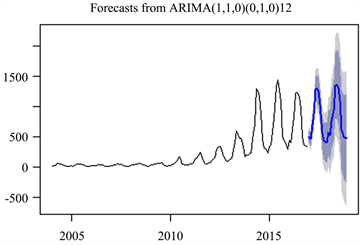Figure 7. The ARIMA(1,1,0)(0,1,0)12 model is proposed to predict the new incidence of human brucellosis in Xinjiang from January to December 2017Table 3. The ARIMA(1,1,0)(0,1,0)12 model with 80% and 95% confidence intervals predicts the number of new cases of human brucellosis in Xinjiang from January 2017 to November 2017Figure 8. The ARIMA(1,1,0)(0,1,0)12 model is proposed to predict the new incidence of human brucellosis in Xinjiang from February to December 2016, and compare with the actual value from February to December 2016Table 4. SARIMA(1,1,0)(0,1,0)12 model predicted and actual values under confidence intervals of 80% and 95%

4. 讨论

NOTES

*通讯作者。

  张艳红. 人畜共患病的流行特点[J]. 畜禽业, 2013(6): 8-9.  Shang, D.Q., Xiao, D.L. and Yin, J.M. (2002) Epidemiology and Control of Brucellosis in China. Veterinary Microbiology, 90, 165-182. https://doi.org/10.1016/S0378-1135(02)00252-3  陈彪, 王涛, 李爱巧, 等. 乌鲁木齐市动物布鲁氏菌病流行病学调查[J]. 中国动物检疫, 2013, 30(3): 28-30.  木合塔尔·艾山, 何海波, 邰新平, 等. 新疆2013年人间布鲁氏菌病监测结果及疫情分析[J]. 中国媒介生物学及控制杂志, 2015, 26(1): 86-88.  潘姣姣, 董柏青, 吕炜, 等. 三种时间序列模型探讨1989~2012广西肺结核发病趋势[J]. 中国卫生统计, 2012, 29(6): 868-870.  陆波, 闵红星, 扈学琴, 等. 时间序列模型预测流感发病率的研究[J]. 中国实用医药, 2014, 9(7): 255-256.  陈纯, 李铁钢, 肖新才, 等. 应用R软件对比两种手足口病发病预测模型的效果[J]. 国际流行病学传染病学杂志, 2016, 43(2): 101-104.  易燕飞. 基于时间序列模型的传染病流行趋势及预测研究[D]: [硕士学位论文]. 长春: 长春工业大学, 2016.  Xu, Q.Q., Li, R.Z., Liu, Y.F., Luo, C., Xu, A.Q., Xue, F.Z., Xu, Q. and Li, X.J. (2017) Forecasting the Incidence of Mumps in Zibo City Based on a SARIMA Model. International Journal of Environmental Research and Public Health, 14, 925. https://doi.org/10.3390/ijerph14080925  汪鹏, 彭颖, 杨小兵. ARIMA模型与Holt-Winters指数平滑模型在武汉市流感样病例预测中的应用[J]. 现代预防医学, 2018, 45(3): 385-389.  吴喜之, 刘苗. 应用时间序列分析[M]. 第2版. 北京: 机械工业出版社, 2018: 38-39.  妥小青, 张占林, 龚政, 等. 基于ARIMAX模型的乌鲁木齐市流感样病例预测分析[J]. 中华疾病控制杂志, 2018, 22(6): 590-593.  Hyndman, R.J. and Khandakar, Y. (2008) Automatic Time Series Forecasting: the forecast Package for R. Statistical Software, 27, 16. https://doi.org/10.18637/jss.v027.i03  漆莉, 李革, 李勤. ARIMA模型在流行性感冒预测中的应用[J]. 第三军医大学学报, 2007, 29(3): 267-269.